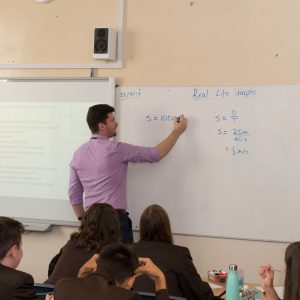# Mathematics

Introduction

In Years 9, 10 and 11 we prepare our students for Mathematics Higher GCSE with the AQA examination board.

What will it be like?

Mathematics builds on knowledge learnt in years 7 and 8. Students will study the following elements:

 Number Ratio, Proportion and Rates of Change Statistics Algebra Geometry and Measures Probability

The structure involves three assessment objectives:

 Assessment Objective What it is What this means % of marks in the exam A01 Recall and use knowledge of mathematics taught Standard questions testing your knowledge of each topic 40 A02 Select and apply mathematical methods in a range of contexts Deciding what method you need to use to get to the correct solution to a problem in context 30 A03 Interpret and analyse problems and generate strategies to solve them. Solve problems by deciding how and explaining why 30Therefore, we place a big emphasis on students’ being analytical in their approach to studying mathematics and being fluent in explaining their methods and reasoning. Appropriate use of a calculator is also important.

Assessment

Students sit three examinations at the end of Year 11, each 1 ½ hours long.  They carry equal weighting for the final mark.  Two are calculator papers and the other is a non-calculator paper.  It is our intention that all our students are entered for Higher tier. The grades available are 4 to 9, with 9 being the highest.

Extra Qualifications in Mathematics

For some students, we also offer one of two offer extra qualifications at the end of year 11. Decisions about who takes these exams will be made at the beginning of year 11.

OCR Level 3 FSMQ Additional Mathematics.  The grades available here are A to E, all of which are pass grades. UCAS points are awarded according to the grade achieved.

AQA Level 2 Further Mathematics.  The grades available here are 9 to 5.